# KSEEB Solutions for Class 9 Maths Chapter 4 Polynomials Ex 4.5

In this chapter, we provide KSEEB SSLC Class 9 Maths Chapter 4 Polynomials Ex 4.5 for English medium students, Which will very helpful for every student in their exams. Students can download the latest KSEEB SSLC Class 9 Maths Chapter 4 Polynomials Ex 4.5 pdf, free KSEEB SSLC Class 9 Maths Chapter 4 Polynomials Ex 4.5 pdf download. Now you will get step by step solution to each question.

## Karnataka Board Class 9 Maths Chapter 4 Polynomials Ex 4.5

KSEEB Solutions For Class 9 Maths Polynomials Question 1.
Use suitable identities to find the following products :
(i) (x + 4) (x + 10)
(ii) (x + 8) (x – 10)
(iii) (3x + 4) (3x – 5)
(iv) (y2+32)(y2−32)
(v) (3 – 2x) (3 + 2x)
Solution:
(i) (x + 4) (x + 10)
Suitable identity is (x + a) (x + b) = x2 + (a + b)x + ab
(x + 4) (x + 10) = x2 + (4 + 10)x + (4 × 10)
= x2 + 14x + 40

(ii) (x + 8) (x- 10)
Suitable identity is (x + a) (x + b) = x2 + (a + b)x + ab
(x + 8) (x – 10)= (x)2 + (8 – 10)x + (8 × -10)
= x2 + (-2)x + (-80)
= x2 – 2x – 80

(iii) (3x + 4) (3x – 5)
Suitable identity is (x + a) (x + b) = x2 + (a + b)x + ab
(3x + 4) (3x – 5) = (3x)2 + (+4 – 5)3x + (4 x -5)
= 9x2 + (-1)3x + (-20)
= 9x2 – 3x – 20

(iv) (y2+32)(y2−32)
Suitable identity is (a + b) (a – b) = a2 – b2
(y2+32)(y2−32)=(y2)2−(32)2=y4−94

(v) (3 – 2x) (3 + 2x)
Suitable identity is (a + b) (a – b) = a2 – b2
(3 – 2x) (3 + 2x) = (3)2 – (2x)2
= 9 – 4x2.

KSEEB Solutions For Class 9 Maths Question 2.
Evaluate the following products without mulitplying directly :
(i) 103 × 107
(ii) 95 × 96
(iii) 104 × 96
Solution:
(i) 103 × 107
= (100 + 3) (100 – 3)
As per Identity,
(a + b)(a – b) = a2 – b2
(100 + 3)(100 – 3) = (100)– (3)2
= 10000 – 9
= 9991

(ii) 95 × 96
= (100 – 5) (100 + 6)
As per Identity.
(x + a) (x + b) = x2 + (a + b)x + ab
(100 – 5) (100 + 6) = (100)+ (-5 + 6)100 + (-5 × 6)
= 10000 + (1)100 + (-30)
= 10000 + 100 – 30
= 10100 – 30
= 10070

(iii) 104 × 96
= (100 + 4) (100 – 4)
As per Identity,
(a + b) (a – b) = a2 – b2
(100 + 4) (100 – 4) = (100)2 – (4)2
= 10000 – 16
= 9984

Exercise 4.5 Class 9 Polynomials Question 3.
Factorise the following using appropriate identities :
(i) 9x2 + 6xy + y2
(ii) 4y2 – 4y + 1
(iii) x2−y2100
Solution:
(i) 9x2 + 6xy + y2
= (3x)2 + 2(3x)(y) + (y)2
= (3x + y)2 [∵ x2 + 2xy + y2 = (x + y)2]

(ii) 4y2 – 4y + 1
= (2y)2 – 2(2y)(1) + (1)2
= (2y – 1)2 [∵ x2 – 2xy + y2 = (x – y)2]

(iii) x2−y2100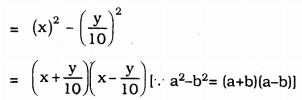9th Maths Polynomials Exercise 4.5 Question 4.
Expand each of the following, using suitable identities :
(i) (x + 2y + 4z)2
(ii) (2x – y + z)2
(iii) (-2x + 3y + 2z)2
(iv) (3a – 7b – c)2
(v) (-2x + 5y – 3z)2
(vi) (14a−12b+1)2
Solution:
(i) (x + 2y + 4z)2
(a + b + c)2 = a2 + b2 + c2 + 2ab + 2bc + 2ca
(x + 2y + 4z)2 =(x)2 + (2y)2 + (4z)2 + 2(x)(2y) + 2(2y)(4z) + 2(4z)(x)
= x2 + 4y2 + 16z2 + 4xy + 16yz + 8zx

(ii) (2x – y + z)2
(x + y + z)2 = x2 + y2 + z2 + 2xy + 2yz + 2zx (2x – y + z)2= (2x)2 + (-y)2 + (z)2 + 2(2x)(-y) + 2(-y)(z) + 2(z)(2x)
= 4x2 + y2 + z2 – 4xy – 2yz + 4zx.

(iii) (-2x + 3y + 2z)2
(x + y + z)2 = x2 + y2 + z2 + 2xy + 2yz + 2zx (-2x + 3y + 2z)2
= (-2x)2 + (3y)22 + (2z)2 + 2(-2x)(3y) + 2(3y)(2z) + 2(2z)(-2x)
= 4x2 + 9x2 + 4z2 – 12xy + 12yz – 8zx

(iv) (3a – 7b – c)2
(a + b + c)2 = a2 + b2 + c2 + 2ab + 2bc + 2ca (3a – 7b – c)2 =(3a)2 + (-7b)2 + (-c)2 + 2(3a)(-7b)
+ 2(-7b)(-c) + 2(-c)(3a) = 9a2 + 49b2 + c2 – 42ab – 14bc – 6ca.

(v) (-2x + 5y – 3z)2
(x + y + z)2 = x2 + y2 + z2 + 2xy + 2yz + 2zx (-2x + 5y – 3z)2 = (-2x)2 + (5y)2 + (-3z)2 + 2(-2x)(5y) + 2(5y)(-3z) + 2(-3z)(-2x) = 4x2 + 25y2 + 9z2 – 20xy – 30yz + 12zx

(vi) (14a−12b+1)2
(a + b + c)2 = a2 + b2 + c2 +2ab + 2bc + 2ca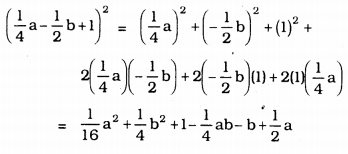Class 9 Maths Chapter 4 Exercise 4.5 Solutions Question 5.
Factorise :
(i) 4x2 + 9y2 + 16z2 + 12xy – 24yz – 16xz
(ii) 2x2 + y2 + 8z2 – 22–√xy + 42–√yz- 8xz
Solution:
(i) 4x2 + 9y2 + 16z2+ 12xy – 24yz – 16xz
= (2x)2+ (3y)2 + (-4z)2 + 2(2x)(3y) + 2(3y)(-4z) + 2(-4z)(2x)
= (2x + 3y – 4z)2.

(ii) 2x2 + y2 + 8z2 – 22–√xy + 42–√yz- 8xz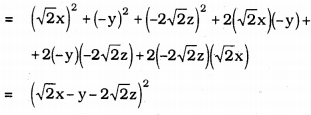KSEEB Solutions For Class 9th Maths Polynomials Question 6.
Write the following cubes in expanded form:
(i) (2x + 1)3
(ii) (2a – 3b)3
(iii) [32x+1]3
(iv) [x−23y]3
Solution:
(i) (2x + 1)3
As per Identity,
(a + b)3 = a3 + b3 + 3ab(a + b)
(2x + 1)3 = (2x)3 + (1)3 + 3(2x)(1)(2x + 1)
= 8x3 + 1 + 6x(2x + 1)
= 8x3 + 1 + 12x2 + 6x
(2x + 1)3 = 8x3 + 12x2 + 6x + 1.

(ii) (2a – 3b)3
As per Identity,
(a – b)3 = a3 – b3 – 3ab(a – b)
(2a – 3b)3= (2a)3– (3b)3 – 3(2a)(3b)(2a-3b)
= 8a3 – 27b3 – 18ab(2a – 3b)
(2a – 3b)3= 8a3 – 27b3 – 36a2b + 54ab2

(iii) [32x+1]3
As per Identity,
(a + b)3 = a3 – b3 – 3ab(a + b)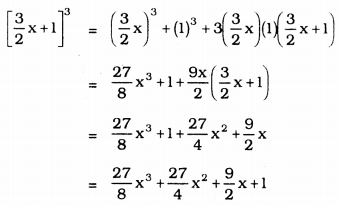(iv) [x−23y]3
As per Identity,
(a – b)3 = a3 – b3 – 3ab(a – b)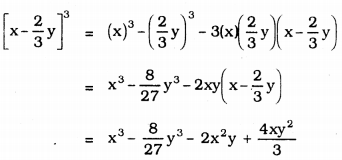KSEEB Solutions For Class 9 Maths Polynomials Exercise 4.5 Question 7.
Evaluate the following using suitable identities :
(i) (99)3
(ii) (102)3
(iii) (998)3
Solution:
(i) (99)3 = (100 – 1)3
As per Identity,
(a – b)3 = a3 – b3 – 3ab(a – b)
(100 – 1)3 = (100)3 – (1)3 – 3(100)(1)(100- 1)
(99)3 = 1000000 – 1 – 300(100 – 1)
= 1000000 – 1 – 30000 + 300
= 970299
∴ (99)3 = 970299

(ii) (102)3 = (100 + 2)3
As per Identity,
(a + b)3 = a3 + b3 + 3ab(a + b)
(100 + 2)3 = (100)3 + (2)3 + 3( 100)(2)( 100 + 2)
= 1000000 + 8 + 600( 100 + 2)
= 1000000 + 8 + 60000 + 1200
(100 + 2)3 = 1061208
∴ (102)3= 1061208

(iii) (998)3 = (1000 – 2)3
As per Identity,
(a – b)3 = a3 – b3 – 3ab(a – b)
(1000 – 2)3 = (1000)3 – (2)3 – 3( 1000) (2)(1000 – 2)
= 1000000000 – 8 – 6000(1000 – 1)
= 1000000000 – 8 – 6000000 + 6000
= 994005992
∴ (998)3 = 994005992

KSEEB Solutions For Class 9 Maths Chapter 4 Question 8.
Factorise each of the following :
(i) 8a3 + b3 + 12a2b + 6ab2
(ii) 8a3 – b3 – 12a2b + 6ab2
(iii) 27- 125a3– 135a + 225a2
(iv) 64a3 – 27b3 – 144a2b + 108ab2
(v) 27p3−1216−92p2+14p
Solution:
(i) 8a3 + b3 + 12a2b + 6ab2
= (2a)3 + (b)3 + 3(2a)(b)(2a + b)
= (2a + b)3 ∵ a3 + b3 + 3ab(a + b) = (a + b)3

(ii) 8a3 – b3 – 12ab2 + 6ab3
= (2a)3 + (-b)3 + 3(2a)(-b)(2a – b)
= (2a – b)3 ∵ a3 – b3 – 3ab(a – b) = (a – b)3

(iii) 27 – 125a3 – 135a + 225a2
OR 125a3 – 225a2 + 135a – 27
= (5a)3 + (-3)3 + 3(5a)(-3)(5a – 3)
= (5a – 3)3 ∵ a3 – b3 – 3ab(a – b) = (a – b)3

(iv) 64a3 – 27b3 – 144a2b + 108ab2
= (4a)3 + (-3b)3 + 3(4a)(-3b)(4a – 3b)
= (4a-3b)3 ∵ a3 – b3 – 3ab(a – b) = (a – b)3

Maths Exercise 4.5 Class 9 Question 9.
Verify :
(i) x3 + y3 = (x + y) (x2 – xy + y2)
(ii) x3 – y3 = (x – y) (x2 + xy + y2)
Solution:
(i) x3 + y3 = (x + y)(x2 – xy + y2)
L.H.S. = x3 + y3
R.H.S. = (x + y)(x2 – xy + y2)
= x(x2 – xy + y2) + y(x2 – xy + y2)
= x3 – x2y + xy2 + x2y – xy2 + y3
R.H.S. = x3 + y3
∴ L.H.S. = R.H.S.
∴ x3 + y3 = (x + y)(x2 – xy + y2)

(ii) x3 – y3 = (x – y)(x2 + xy + y2)
L.H.S. = x3 – y3
R.H.S. = (x – y)(x2 + xy + y2)
= x(x2 + xy + y2) – y(x2 + xy + y2)
= x3 + x2y + xy2 – x2y – xy3 – y3
R.H.S. = x3 – y3
∴L.H.S. = R.H.S.
∴ x3 – y3 = (x – y)(x2 + xy + y2)

Polynomials Class 9 KSEEB Solutions Question 10.
Factorise each of the following :
(i) 27y3 + 125z3
(ii) 64m3 – 343n3
[Hint: See Question 9).
Solution:
(i) 27y3 + 125z3
= (3y)3 + (5z)3
= (3y + 5z) {(3y)2 – (3y)(5z) + (5z)2}
= (3y + 5z) (9y2 – 15yz + 25z2)

(ii) 64m3 – 343n3
= (4m)3 – (7n)3
= (4m – 7n) {(4m)2 + (4m) (7n) + (7n)2}
= (4m – 7n) (17m2 + 28mn + 49n2)

Question 11.
Factorise : 27x3 + y3 + z3 – 9xyz
Solution:
27x3 + y3 + z3 – 9xyz
= (3x)3 + (y)33 + (z)3 – 3(3x)(y)(z)
= (3x + y + z) {(3x)2 + (y)2 + (z)2 – (3x)(y) – (y)(z) – (z)(3x)}
= (3x + y + z) (9x2 + y2 + z2 – 3xy – yz – 3zx)

KSEEB Maths Solutions For Class 9 Question 12.
Verify that x3 + y3 + z3 – 3xyz = 12 (x + y + z)[(x – y)2 + (y – z)2 + (z – x)2]
Solution:
L.H.S.= x3 + y3+ z3 – 3xyz
R.H.S. = 12 (x+y+z) [(x – y)2 + (y – z)2 + (z – x)2]
= 12 (x+y+z) [x2 2xy + y2+ y2 – 2yz + z2 + z2 -2zx + x2]
= 12 (x+y+z) [2x2+ 2y2+ 2z2– 2xy – 2yz – 2zx]
= 12 (x+y+z) × (x2 + y2 + z2 – xy – yz – zx)
= (x + y + z)(x2 + y2 + z2 – xy – yz – zx)
R.H.S.= x3 + y3 + z3 – 3xyz
∴ L.H.S. = R.H.S.
∴ x3 + y3 + z3 – 3xyz = 12 (x + y + z)[(x – y)2 + (y – z)2 + (z – x)3]

9th Standard Maths Exercise 4.5 Question 13.
If x + y + z = 0, show that x3 + y3 + z3 = 3xyz.
Solution:
x + y + z = 0
x + y = -z
by cubing on both sides,
(x + y)3 = (-z)3
x3+ y3 + 3xy(x + y) = -z3
x3 + y3 + 3xy (-z) = -z3
(∵ x + y =-z data given)
x3 + y3 – 3xyz = -z3
∴ x3 + y3 + z3 = 3xyz.

KSEEB Solutions For Class 9th Maths Question 14.
Without actually calculating the cubes, find the value of each of the following:
(i) (-12)3 + (7)3 + (5)3
(ii) (28)3 + (-15)3 + (-13)3
Solution:
(i) (-12)3 + (7)3 + (5)3
Let -12 = x, 7 = y, 5 = z, then
x3 + y3 + z3 = 3xyz.
∴ (-12)3 + (7)3 + (5)3
= 3(-12)(7)(5)
= -36 × 35
= -1260.

(ii) (28)3 + (-15)3 + (-13)3
Let x = 28, y = -15, z = -13, then
x3 + y3 + z3 = 3xyz
∴ (28)3 + (-15)3 + (-13)3
= 3(28)(- 15)(-13)
= 84 × 195
= 16380

KSEEB 9th Maths Solutions Question 15.
Give possible expressions for the length and breadth of each of the following rectangles, in which their areas are given :
(i) Area: 25a2 – 35a +12
(ii) Area : 35y2 + 13y – 12
Solution:
(i) Area: 25a2 – 35a + 12
= 25a2 – 20a – 15a + 12
= 5a(5a – 4) – 3(5a – 4)
= (5a -4) (5a – 3)
length × breadth = Area of rectangle
(5a – 4) (5a – 3) = 25a2 – 35a + 12
∴ Length = (5a – 4)

(ii) Area : 35y2 + 13y – 12
Area of Rectangle – Length × Breadth
35y2 + 13y – 12
35y2 + 28y – 15y
7y(5y + 4) – 3(5y + 4)
(7y – 3) (5y + 4)
∴ Length = (7y – 3)

KSEEB Solutions Class 9 Maths Question 16.
What are the possible expressions for the dimensions of the cuboids whose volumes are given below :
(i) Volume: 3x2 – 12x
(ii) Volume: 12ky2 + 8ky – 20k
Solution:
(i) length × breadth × height = Volume of Cuboid
= 3x2 – 12x
= 3x2 – 12x
= 3x(x – 4)
= 3 × x × (x – 4)
l × b × h
∴ Length = 3
Height = (x – 4)

(ii) length × breadth × height = Volume of Cuboid
= 12ky2 + 8ky – 20k
12ky2 + 8ky – 20k
= 4k(3y2 + 2y – 5)
= 4k (3y2 + 5y – 3y – 5)
= 4k (y(3y + 5) – 1 (3y + 5))
= 4k × (3y + 5) × (y – 1)
= l × b × h
∴ Length = 4k
Height = (y – 1)

All Chapter KSEEB Solutions For Class 9 Maths

—————————————————————————–

All Subject KSEEB Solutions For Class 9

*************************************************

I think you got complete solutions for this chapter. If You have any queries regarding this chapter, please comment on the below section our subject teacher will answer you. We tried our best to give complete solutions so you got good marks in your exam.

If these solutions have helped you, you can also share kseebsolutionsfor.com to your friends.

Best of Luck!!